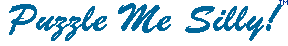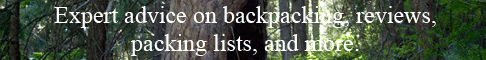Cynical Humor 3 ``` 13. V R Q B G A : M W V A C G T V S W K G J V R H J M T V L C W M W V A , L D G C V H I C Q T V C C Q B Q C B G C V W M A M B V B D V L D V M B U A G S B D V E D M U U , M T R B G C V V B D M B B D V E D M U U Q C W A Q T B V R . V K H V A B D I H H M A R   14. V Y V L W A M I W Y V W J W A X G A Z W M Z Y W V R A X W Y M R P S T R G S W P M V R P A M N M F M S W A M Z S M F M A W Z W G D W A X G R M T S P M J W S R F W R A E . V R N W W N , V A X V R L A X G A Z W M Z Y W O G R A Z W G D W E M F T D X A X G A M R W M K A X W E W N G H E P M J W S R F W R A E X G N I W A A W S P W A M T A M K A X W O G H G R N Y W A A X W F X G J W V A . N O V P X A N . W V E W R X M O W S 15. H W M B Q - X T G Q D Z D W X Q O D W G I J Q O J Q R I F V Q H P M W I F D U G D K Z D G , J Q O V I V G I V W D G M U D P M W A F V K O W D Q I M C D J K B J R G J Q O P M W D Z D W D E T K J V Q V Q H I F V Q H G I M I F D U . J Q I M V Q D O D G J V Q I - D E X T D W R , " I F D K V I I K D T W V Q A D "   16. P ' W G O M B G P B J M C D B A L G A E M N [ N M L J D B G - J G T T G L ' E S X L T M L E ] , X B A C X W G I G G B J M T A J C M D E X B A E M N J C P B O E , I D J B M I M A F G W G L J M T A K G P Q X E X S M T P U G Q M K X B O G J J P B O L G X A F J M X L L G E J C G L . B G Q F M L Z U P J F A G J G U J P W G ``` ```  17. T O T M B J I L C T R J F F K H H V K F J M G C P K N V L C N Q O I J F F T H V J M E V J C N M L C C V L M L M X , O N E H T M E C L E O T Z B E V J Q L M N E J L E O J X L M C E K I T L M . Q T I B E H T L M ( 1 8 3 5 - 1 9 1 0 ) ``` ```  18. Q R Q I E P M I E D Q L T P E J T F Y Q K B S U D W F A M R M A Q A M I E B E D B P Q G D M O D L K B Y T Y W F I Q R Q K D T L L Q I Q A T I A E D B P Q G D M O D A B I B E J T E E Q K . G . K . M I U Q ``` Copyright © 2012 Variety Games Inc.